Student Learning Objectives
Lessons / Lecture Notes
Important Equations
Example Problems
Applets and Animations

Student Learning Objectives

• To understand the size, structure, and composition of the nucleus.
• To learn about the properties of the strong force.
• To understand the forces that hold the nucleus together and under what circumstances it might break apart.
• Develop a basic understanding of some applications of nuclear physics and an appreciation of some of the principles of particle physics.
• To understand radioactive decay and half-lives.
• To distinguish between the different types of radioactive decays and compute the daughter nuclei for these decays.
• Use the concept of half-life to determine the age of a radioactive sample.

Lessons / Lecture Notes

PY106 Notes from Boston University (algebra-based):

Physics 2C notes from Dr. Bobby W.S. Lau (algebra-based)

HyperPhysics (calculus-based)

Important Equations (for algebra-based Physics)

Example Problems

Example Problems for algebra-based physics (from College Physics 2nd Edition by Knight, Jones, and Field & Physics 8th Edition by Cutnell and Johnson):

Example Problems (Nuclear Physics)
Solutions to Example Problems (Nuclear Physics)

Applets and Animations

 Alpha Decay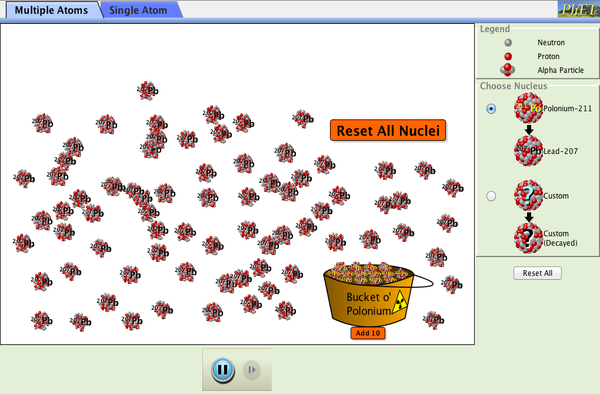Watch alpha particles escape from a polonium nucleus, causing radioactive alpha decay. See how random decay times relate to the half life. Beta DecayWatch beta decay occur for a collection of nuclei or for an individual nucleus. Radiation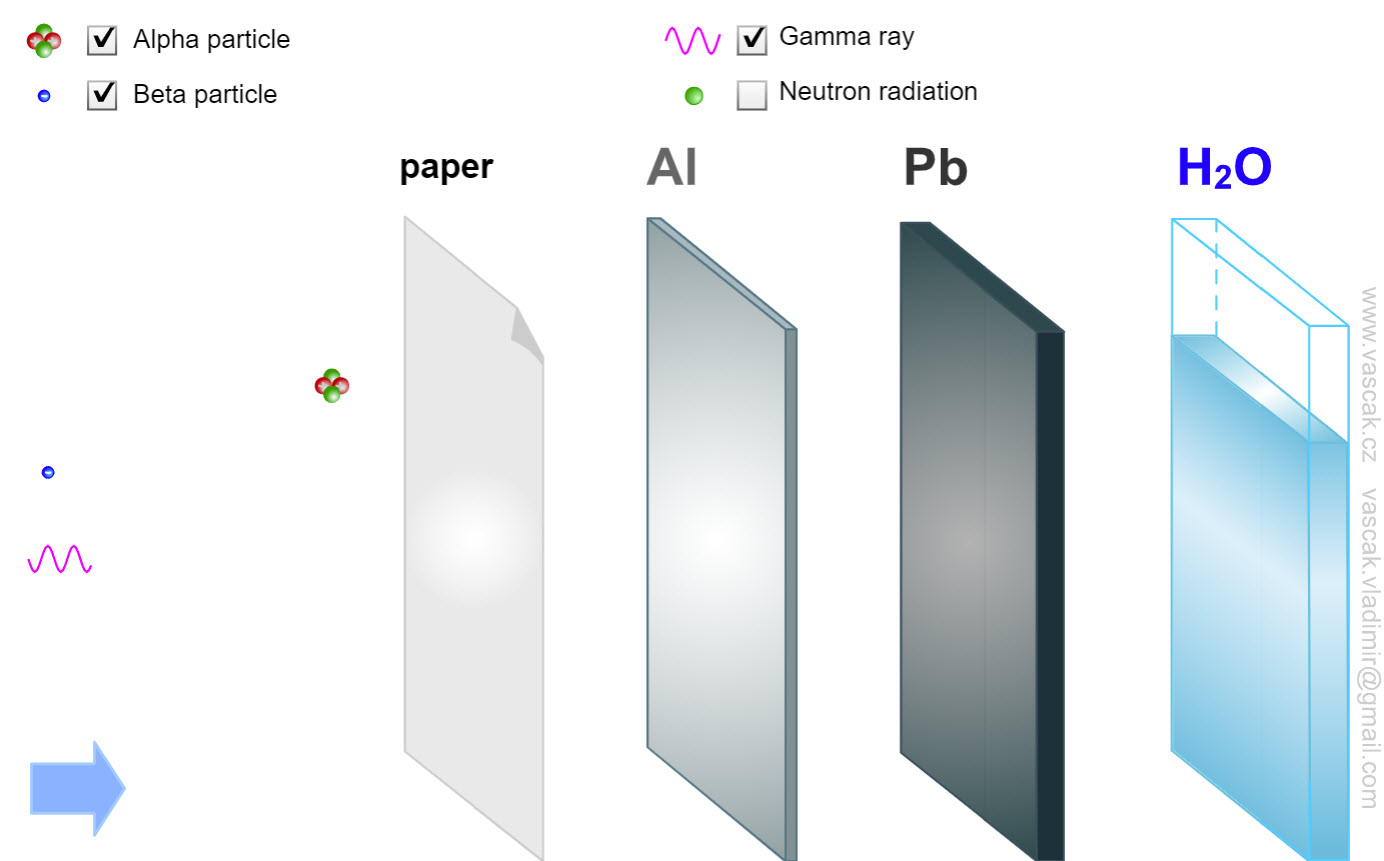Nice animation showing the different types of radiation. Nuclear Reactions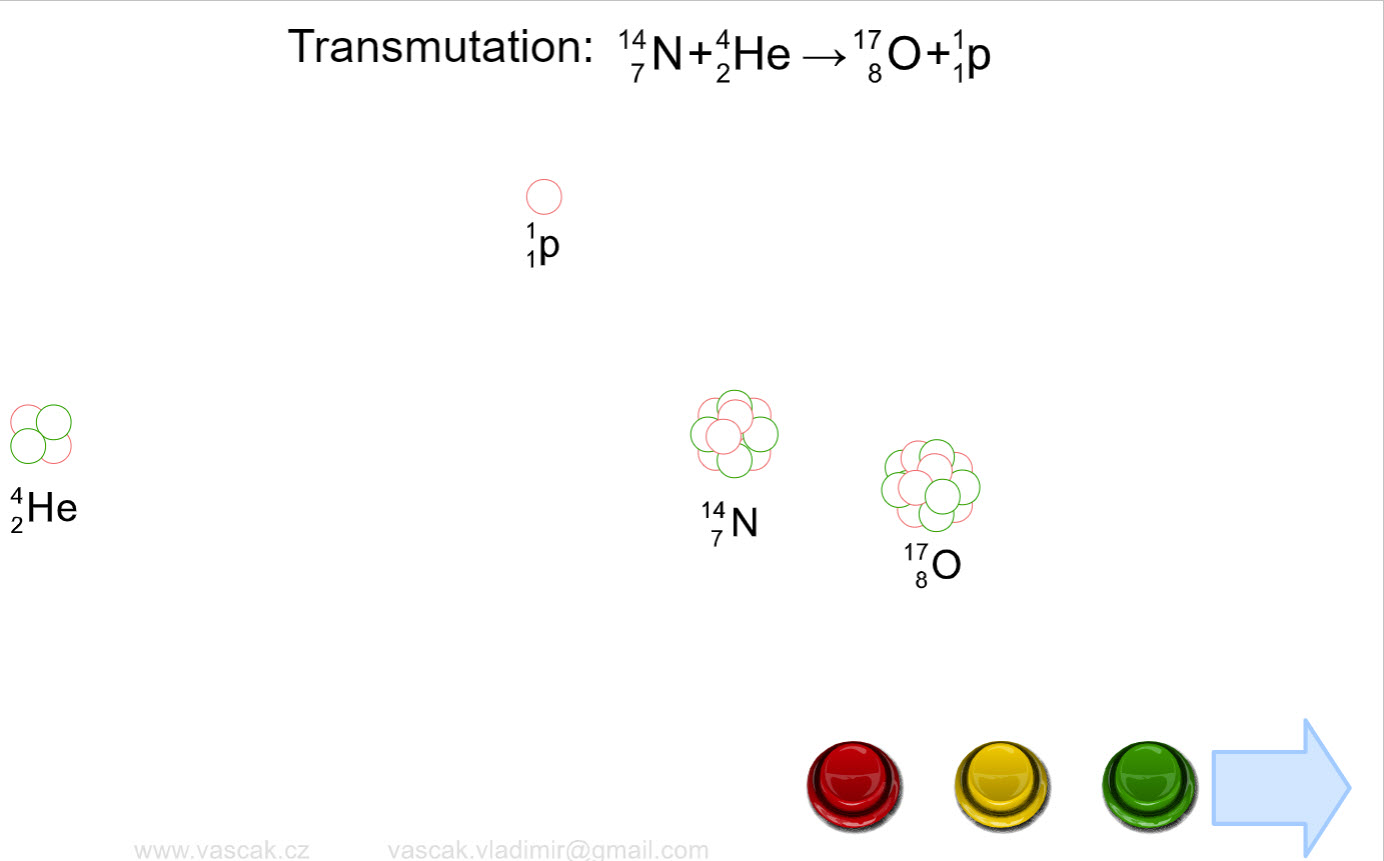Nice animation showing the different types of nuclear reactions. Radioactive Decay Chain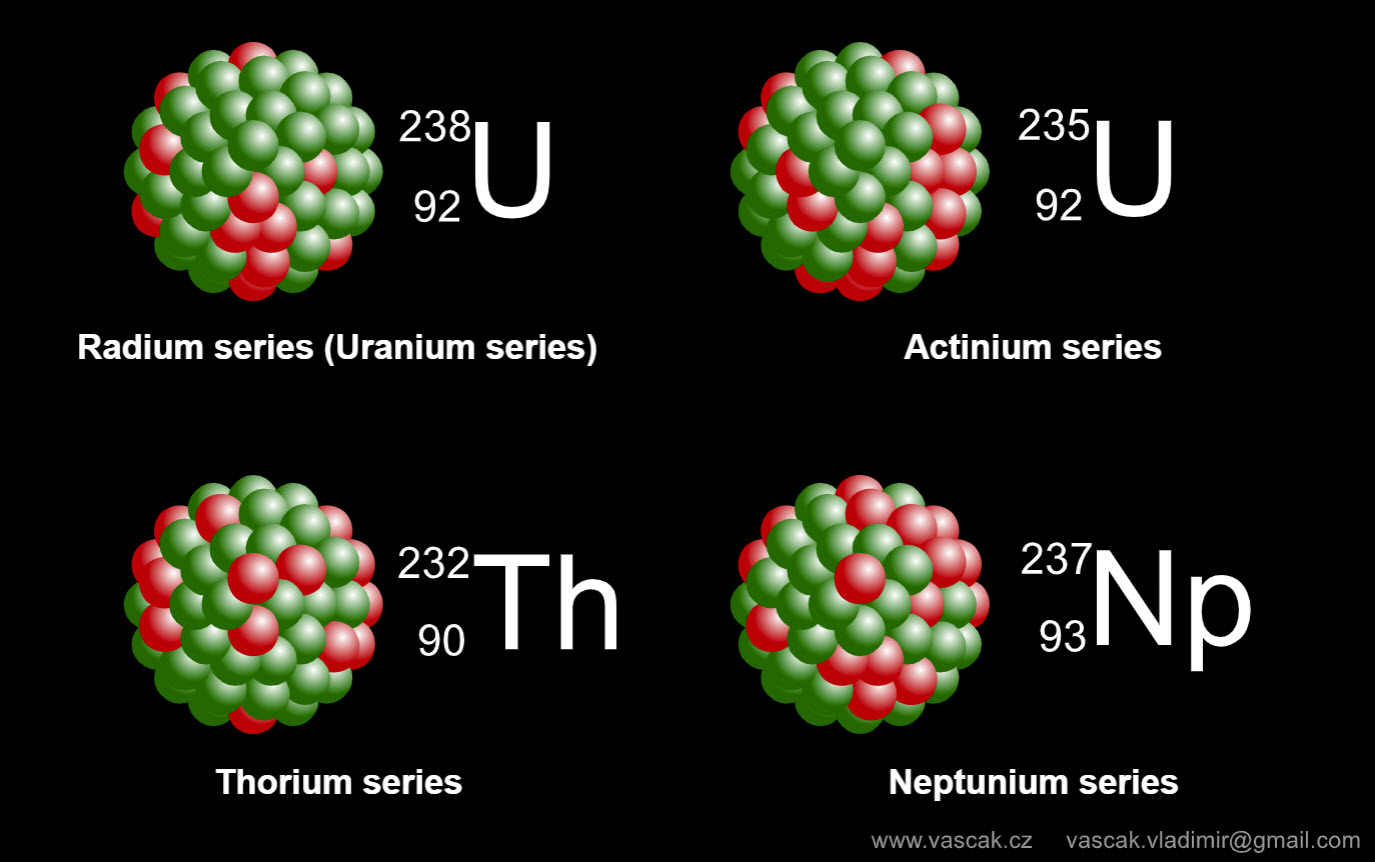Nice animation showing the different types of radioactive decay chains. Radioactive Decay Chains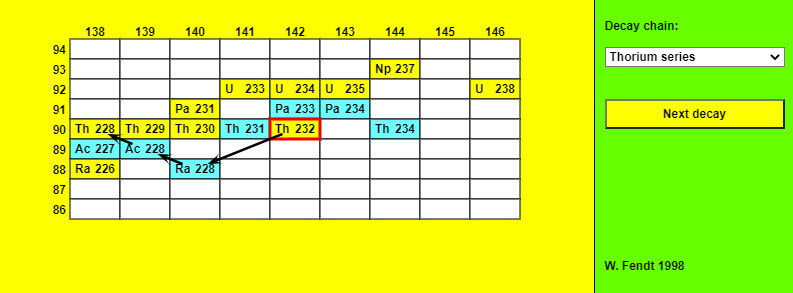This app shows a small part of the table of nuclids in which short notations like Th 232 (consisting of the chemical symbol and the mass number) are used for reasons of space. The number of protons is indicated at the left, the number of neutrons at the beginning of a column. Yellow color is used for alpha decay, blue color for beta decay. After you have chosen one of the decay chains in the selection field on the top right, you can see by repeated mouse clicks on the yellow button ("Next decay") which nuclei are formed one after the other. Chain ReactionA nucleus in the middle emits a neutron in a random direction, if it hits another nucleus it is absorbed, that hit nucleus emits two neutrons and dies. A full chain reaction, involving essentially all the nuclei, is much more likely to occur with a bigger piece of material. In real life, with trillions of trillions of nuclei (at least) there is a precise critical size (for a given shape) at which the reaction "goes critical" and explodes. With our far smaller grids the random fluctuations are large, so we can just get the general idea. For the smallest grid, you'll find most times not much happens. For the largest grid, almost all the nuclei will explode. Try it! The mean free path is how far the neutron gets on average before hitting another nucleus. Roughly it's the inverse of the ratio of the nuclear size (the 'cross section") to the atom size (meaning distance between nuclei). This is how many rows of atoms the neutron will get through, on average, before it hits a nucleus. For a real neutron in uranium, for example, the cross section of the nucleus is (now two-dimensional) less than a billionth the size of the atom, so the neutron can go through billions of rows (actually planes, in 3D) of atome, the mean free path is of order centimeters. This gives the scale of size needed for a successful chain reaction. The Law of Radioactive Decay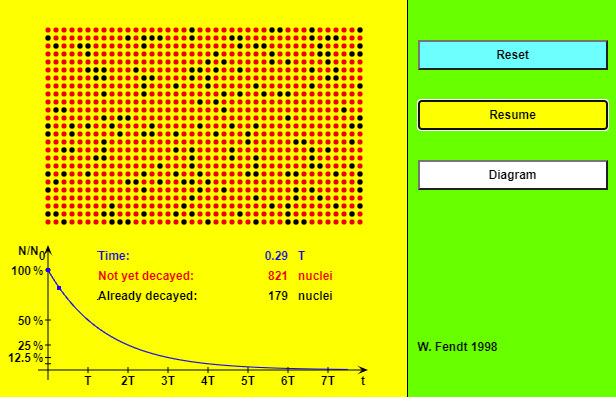As soon as the app is started with the yellow button, the atomic nuclei will begin to "decay" (change of color from red to black). You can stop and continue the simulation by using the button "Pause / Resume". In this case a blue point for the time and the fraction of the not yet decayed nuclei is drawn into the diagram. (Note that often these points don't lie exactly on the curve!) If you want to restore the initial state, you will have to click on the "Reset" button. It is possible to give the probability that a single atomic nucleus will "survive" during a given interval. This probability amounts to 50 % for one half-life period. In an interval twice as long (2 T) the nucleus survives only with a 25 % probability (half of 50 %), in an interval of three half-life periods (3 T) only with 12.5 % (half of 25 %) and so on. You can't, however, predict the time at which a given atomic nucleus will decay. For example, even if the probability of a decay within the next second is 99 %, it is nevertheless possible (but improbable) that the nucleus decays after millions of years. Half Life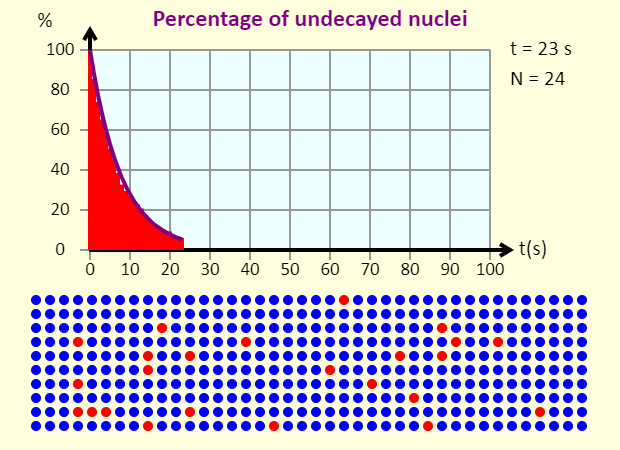This is a simulation of the radioactive decay of 400 radioactive nuclei. You can choose from three different half lives. Note that the nuclei turn blue when they have decayed, and the smooth purple line on the graph shows the ideal case. An actual case can deviate a bit from the ideal case because of statistical fluctuations (which make more of a difference when the number of nuclei is small, as it is here). See if you can estimate the half life in the three cases shown here. Law of Radioactive DecayNice animation showing the law of radioactive decay. Nuclear FissionStart a chain reaction, or introduce non-radioactive isotopes to prevent one. Control energy production in a nuclear reactor! (Previously part of the Nuclear Physics simulation - now there are separate Alpha Decay and Nuclear Fission sims.) Radioactive Dating Game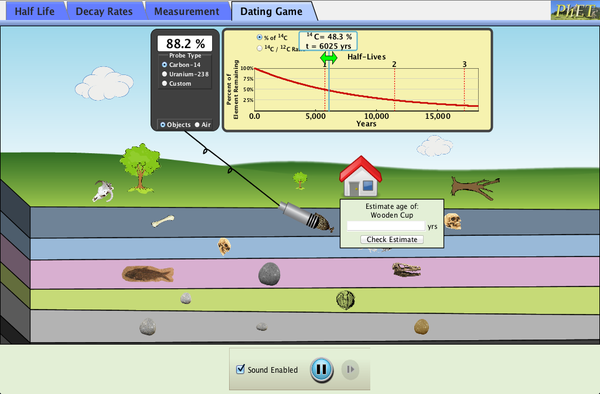Learn about different types of radiometric dating, such as carbon dating. Understand how decay and half life work to enable radiometric dating. Play a game that tests your ability to match the percentage of the dating element that remains to the age of the object.